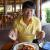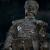为 Java 程序员准备的 10 分钟 Perl 教程已翻译 100%

oschina 投递于 2013/11/08 08:53 (共 4 段, 翻译完成于 11-09)

参与翻译 (3人) : cmy00cmy, 砖家, 为梦而来

1.从基础开始

# comment starts with "#"
# the name is hello.pl
print "Hello Perl!";
perl hello.pl

2. 日期类型

#claim a hash and assign some values
my %aHash;
\$aHash{'a'}=0;
\$aHash{'b'}=1;
\$aHash{'c'}=2;
\$aHash{'d'}=3;
\$aHash{'e'}=4;

#put all keys to an array
my @anArray = keys (%aHash);

#loop array and output each scalar
foreach my \$aScalar (@anArray){
print \$aScalar."\n";
}

e
c
a
b
d

foreach my \$aScalar (sort @anArray){
print \$aScalar."\n";
}

3. 条件、循环表达式

Perl为条件和循环语句准备了if, while, for, foreach等关键字，这与Java非常类似（switch除外）。

#if my \$condition = 0;
if( \$condition == 0){
print "=0\n";
}
elsif(\$condition == 1){
print "=1\n";
}
else{
print "others\n";
}

#while while(\$condition < 5){
print \$condition;
\$condition++;
}
for(my \$i=0; \$i< 5; \$i++){
print \$i;
}

#foreach my @anArray = ("a", 1, 'c');
foreach my \$aScalar (sort @anArray){
print \$aScalar."\n";
}

4.文件的读写

#read from a file
my \$file = "input.txt";
open(my \$fh, "<", \$file) or die "cannot open < \$file!";
while ( my \$aline = <\$fh> ) {
#chomp so no new line character
chomp(\$aline);
print \$aline;
}

close \$fh;

# write to a file
my \$output = "output.txt";
open (my \$fhOutput, ">", \$output) or die("Error: Cannot open \$output file!");
print \$fhOutput "something";
close \$fhOutput;

5.正则表达式

Perl中有两种使用正则表达式的方法：m和s。

\$str =~ m/program(creek|river)/

6.传值/引用的语法

my @testArray = (1, 3, 2);

#In sub sub processArrayByReference(\$) {
my \$arrayref = shift;
my @array    = @\$arrayref;
#...
}

#In sub processarray: sub processArrayByValue(\$){
my @array = @_;
#...
}

processArrayByValue(@testArray);
processArrayByReference( \@testArray );

sub printHash(\$) {
my %hash = %{ shift() };
for my \$key ( sort keys %hash ) {
my \$value = \$hash{\$key};
print "\$key => \$value\n";
}
}

printHash(\%twoLettersCount);

7.一些实例

1).遍历字符串中的每个字符。

my @lineCharArray = split('',\$aline);
foreach my \$character (@lineCharArray){
print \$character."\n";
}

2).创建一个包含26个字母的数组。

my @charArray = ('a'..'z' );
my @twoCharArray = ('aa'..'zz');

评论(17)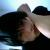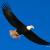引用来自“accacc”的评论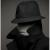引用来自“wxianfeng”的评论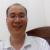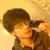引用来自“齐天大圣”的评论